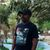# QlikView App Dev

Discussion Board for collaboration related to QlikView App Development.

Announcements
Live chat with experts, bring your API Integration questions. June 15th, 10 AM ET. REGISTER TODAY
cancel
Showing results for
Search instead for
Did you mean:Creator

## Date Variable Set Analysis

Help please,

I need to subtract one date from another (which is fine)

But the date that i am subtracting from is in a variable (vPeriodEnd), and my expression isn't working (it works fine with a standard field, its the variable that is causing me the headache). The date held within the variable is ok, as is the format.

any ideas?

if(

only({<

month=

,year=

,absence = {'Sickness'}

,census_date={'>=\$(=\$(vPeriodStart))   <=\$(=\$(vPeriodEnd))'}

>}\$(vPeriodEnd))

-

only({<

month=

,year=

,absence = {'Sickness'}

,census_date={'>=\$(=\$(vPeriodStart))   <=\$(=\$(vPeriodEnd))'}

>}start_date)

> 28, 'Yes', 'No')

11 RepliesMVP

What exactly is behind vPeriodEnd? Is it an expression or a hard coded value?Creator III

Please try the below expression.

if(

only({<

month=

,year=

,absence = {'Sickness'}

,census_date={">=\$(=vPeriodStart)   <=\$(=vPeriodEnd)"}

>}\$(vPeriodEnd))

-

only({<

month=

,year=

,absence = {'Sickness'}

,census_date={">=\$(=vPeriodStart)   <=\$(=vPeriodEnd)"}

>}start_date)

> 28, 'Yes', 'No')Creator
Author

a date (dd/mm/yyyy)

the user gets to select an end date using a slider on the dashboard to choose the period that they wish to view.

so essentially im subtracting vPeriodEnd - the database field start_dateCreator
Author

Thanks Sibin,

tried that already, doesnt work.MVP

So, via a slider a value is set for the date? May be see if this works

if(

only({<

month=

,year=

,absence = {'Sickness'}

,census_date={'>=\$(=\$(vPeriodStart))  <=\$(=\$(vPeriodEnd))'}

>} Date#(\$(vPeriodEnd), 'DD/MM/YYYY'))

-

only({<

month=

,year=

,absence = {'Sickness'}

,census_date={'>=\$(=\$(vPeriodStart))  <=\$(=\$(vPeriodEnd))'}

>}start_date)

> 28, 'Yes', 'No')Champion III

May be give it a try: using sunny's formatting around your variable in single quotes?

if(

only({<

month=

,year=

,absence = {'Sickness'}

,census_date={'>=\$(=\$(vPeriodStart))   <=\$(=\$(vPeriodEnd))'}

>} Date#('\$(vPeriodEnd)', 'DD/MM/YYYY'))Creator
Author

No, still not working. Proper head scratcher this one.MVP

Would you be able to share a sample to show what exactly you are doing?Master

Is Variable declarations of: vPeriodStart, vPeriodEnd matched with Census_date?